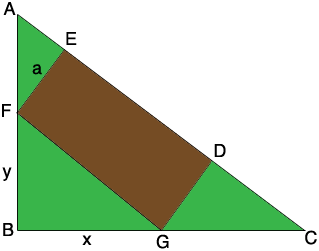SEARCH HOMEMath Central Quandaries & QueriesQuestion from shirlyn, a student: A rectangular building will be constructed on a lot in the form of a right triangle with legs of 60 ft. and 80 ft. If the building has one side along the hypotenuse, find its dimensions for maximum floor area.Hi Shirlyn,

Here is my diagram.|AB| = 60 ft, |BC| = 80 ft, |FB| = y ft, |BG| = x ft and |EF| = a ft. Since triangles ABC and FBG are similar 60/80 = y/x and hence y = 3/4 x.

|AF| = 60 - y ft and Pythagoras theorem tells us that |FG| = √(x2 + y2) ft. Triangles AFE and FBG are similar which allows you to find an expression for a × √(x2 + y2) in terms of x and y. But a × √(x2 + y2) is the area of the rectangle EFGD and now you can use the fact that y = 3/4 x to write the area of the rectangle in terms of x alone. Your knowledge of calculus now allows you to complete the problem.

PennyMath Central is supported by the University of Regina and The Pacific Institute for the Mathematical Sciences.